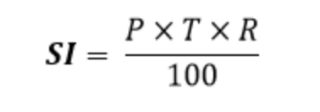Lompat ke konten Lompat ke sidebar Lompat ke footer

# How do I write a C program to calculate Simple Interest?Simple Interest formulaFormula

Where,
P is the principal amount
T is the time and
R is the rate

Video:

Code:

Keep Coding and Keep Learning 🙂